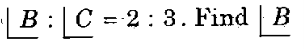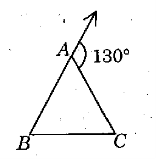# Area and Volume TNPSC Group 1 Questions

## Area and Volume MCQ Questions

7.

Which of the following set of measurements will form a right angle triangle?

Group 4 - 2016

A.

6, 9, 12

B.

5, 8, 10

C.

5, 5, 5√2

D.

3, 1, 4√2

C. 5, 5, 5√2

8.

Three angles of a triangle are x- 30°, x- 45°, x + 15°, find the value of x.

Group 4 - 2016

A.

60°

B.

40°

C.

80°

D.

100°

C. 80°

9.

Which one of the following cannot be the sides of a triangle?

Group 1 - 2019

A.

4,5,6

B.

3,4,5

C.

2,3,4

D.

1,2,3

D. 1,2,3

10.

In a right triangular ground, the sides adjacent to the right angle are 50 m and 80 m. Find the cost of cementing the ground at Rs.5/sq.m

Group 1 - 2019

A.

₹ 20,000

B.

₹ 15,000

C.

₹ 10,000

D.

₹ 12,000

C. ₹ 10,000

11.

Find the percentage increase in the area of a triangle if its each side is doubled.

Group 1 - 2015

A.

100%

B.

200%

C.

300%

D.

400%

C. 300%

12.

In the given figure,Group 4 - 2014

A.

120⁰

B.

52⁰

C.

72⁰

D.

130⁰